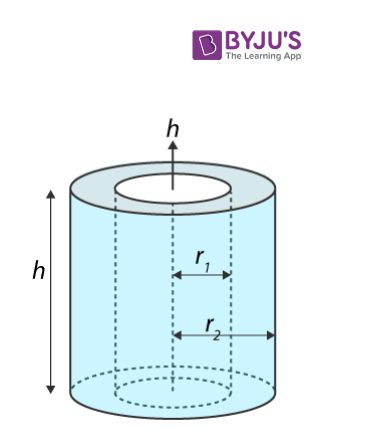Checkout JEE MAINS 2022 Question Paper Analysis : Checkout JEE MAINS 2022 Question Paper Analysis :

# Moment Of Inertia Of A Hollow Cylinder

Moment of inertia of a hollow cylinder that is rotating on an axis passing through the centre of the cylinder where it has an internal radius r1 and external radius r2 with mass M can be expressed in the following manner.

 I = ½ M (r22 + r12)

Subsequently, the moment of inertia of a hollow cylinder with a thin wall is determined using the following equation;

 I = Mr2

Here, the axis goes through the centre of the cylinder and M = mass and r = radius.

## Calculating Moment Of Inertia Of A Hollow Cylinder

If we take a hollow cylinder it will consist of inner radius r1 and outer radius r2 with mass M, and length L. We will calculate its moment of inertia about the central axis.However, before we get into the derivation we have to be aware of certain things. These are;

• The cylinder is split into infinitesimally thin rings.
• Each ring will have a thickness of dr with length L.

Meanwhile, the whole calculation process involves summing up the moments of infinitesimally thin cylindrical shells. It is quite similar to the derivation of the moment of inertia of a solid cylinder.

Let’s begin.

1. First, let us recall the moment of inertia equation:

dI = r2 dm

Here we have to find dm. It is given as;

dm = ρ dV

Since we have mentioned dV in the above equation, we have to calculate it. It will be given as;

dV = dA h

Here the dA is considered as the area of the ring on top. Now we get;

dA = π (r + dr)2 – π r2

dA = π (r2 + 2rdr + (dr)2) – π r2

Here, (dr)2 = 0.

dA = 2 π r dr

We can also find dA by differentiating method.

A = π r2

If we differentiate wrt r,

dA = 2 π r dr

2. We have to substitute dA into dV.

dV = 2 π r h dr

If we substitute into dm we get;

dm = ρ 2 π r h dr

We then substitute the dm expression into the dI equation.

dI = r2 ρ 2 π r hdr

dl = ρ 2 π r3 h dr

3. Now, we can find the expression for density. We use the equation;

ρ = M/V

Now,

ρ = M / hπ(r22 – r12)

4. The next step involves using integration to find the moment of inertia. The integration basically occurs from the inner radius to the outer radius:

dl = ρ 2 π r3 h dr

I = 2 ρ π h r1r2 r3 dr = 2 ρ π h [r24/ 4 – r14/ 4]

=  (ρ π h/2) [r24– r14]

I =(π h/2) [ M / π (r22 – r22) h]  [ (r22 – r12) (r22 + r12)]

I = ½ M (r22 + r12)

Now, if we take a thin hollow cylinder, setting r = r1 = r2 and replacing it into the first equation we get;

I = Mr2

### ⇒ Check Other Object’s Moment of Inertia:

Test Your Knowledge On Moment Of Inertia Of A Hollow Cylinder!